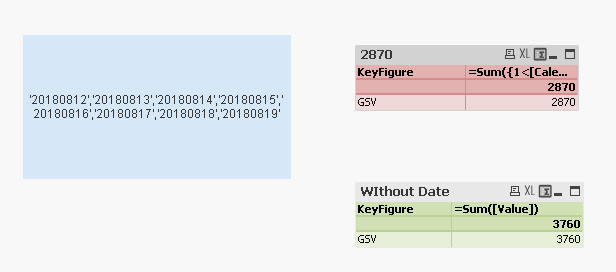# QlikView Scripting

Discussion Board for collaboration on QlikView Scripting.

Announcements
Modernize Your QlikView Deployment webinar, Nov. 3rd. REGISTER
cancel
Showing results for
Did you mean:
HighlightedContributor III

## QlikView Expression - Error in set modifier ad hoc element list: ',' or ')' expected

Hello all,

I'm using an expression in QlikView for a series of values from a field. In this period of the year, currently we have the below days.

'20180812','20180813','20180814','20180815','20180816','20180817','20180818','20180820','20180821','20180822','20180823','20180824','20180827','20180828','20180829','20180830','20180831','20180903','20180904','20180905','20180906','20180907'

Out of which, I need days until yesterday, (assume today is 20180820)

'20180812','20180813','20180814','20180815','20180816','20180817','20180818'

So I used the below script, and it works as expected, by using a variable - \$(vCurPeriodDatesSep_UntilYesterday)

Chr(39)

&

LEFT(REPLACE(

CONCAT({<PeriodInd = {\$(vCurPeriodIndStatic)},

[Calendar Date] = P([Calendar Date])>}distinct [Calendar Date], '*,*')

,

'*',

Chr(39))

,

INDEX(REPLACE(

CONCAT({<PeriodInd = {\$(vCurPeriodIndStatic)},

[Calendar Date] = P([Calendar Date])>}distinct [Calendar Date], '*,*')

,

'*',

Chr(39)), Date(Today(), 'YYYYMMDD'))-3)

But when I'm using the same in a table within Set Analysis like shown below, it shows blank and mentions the error - Error in set modifier ad hoc element list: ',' or ')' expected

[Calendar Date] = {\$(vCurPeriodDatesSep_UntilYesterday)},

PeriodInd = {\$(vCurPeriodIndStatic)},

Country = {'\$(vCountrySelected)'},

[KeyFigure] = {'\$(vCurSAKeyFigure)'}>}[Value])

Thanks and regards,
Ambareesh Jonnavittula

1 Solution

Accepted Solutions
HighlightedMVP

Try this

=Sum({1<[Calendar Date] = {\$(=\$(vCurPeriodDatesSep_UntilYesterday))}>}[Value])6 Replies
HighlightedMVP

Would you be able to share a sample where we can see the issue?

HighlightedContributor III

Hello Sunny, thank you for your quick response. Please see the attachment scenario.

HighlightedMVP

Try this

=Sum({1<[Calendar Date] = {\$(=\$(vCurPeriodDatesSep_UntilYesterday))}>}[Value])HighlightedMaster II

=Chr(39)

&

LEFT(REPLACE(

CONCAT({<

[Calendar Date] = P([Calendar Date])>}distinct [Calendar Date], '*,*')

,

'*',

Chr(39))

,

INDEX(REPLACE(

CONCAT({<

[Calendar Date] = P([Calendar Date])>}distinct [Calendar Date], '*,*')

,

'*',

Chr(39)), Date(Today(), 'YYYYMMDD'))-3)

HighlightedContributor III

Thank you both. The issue is resolved.HighlightedChampion III

Do you always calculate the expression until yesterday then may be you can also use like

=Sum({<

[Calendar Date] = {"<\$(=Date(Today(), 'YYYYMMDD'))" }>}[Value])